Next:1.3 To Probe FurtherUp:1.2 Complexity of AlgorithmsPrevious:1.2.6 Big Omega and Big Theta Notations

## 1.2.7 An Example:

Let f (n) = 2n2 + 4n + 10. f (n) is O(n2). For,

f (n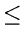3n2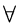n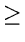6

Thus, C = 3 and n0 = 6

Also,

f (n4n2n4

Thus, C = 4 and n0 = 4

f (n) is O(n3)

In fact, if f (n) is O(nk) for some k, it is O(nh) for h > k

f (n) is not O(n).

Suppose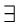a constant C such that
2n2 + 4n + 10Cnnn0
This can be easily seen to lead to a contradiction. Thus, we have that:

f (n) is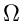(n2) and f (n) is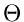(n2)

eEL,CSA_Dept,IISc,Bangalore